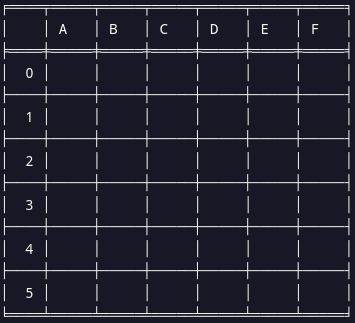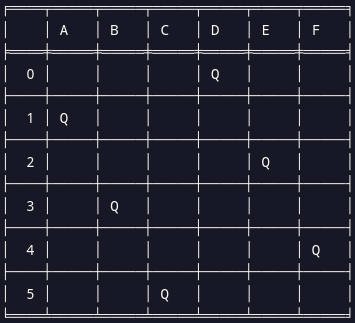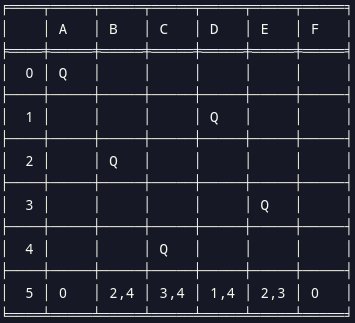×

Search anything:

# Backjumping

#### backtracking AlgorithmsGet this book -> Problems on Array: For Interviews and Competitive Programming

In this article, we have covered the concept of Backjumping which is an improvement to the Backtracking Algorithm. We will solve the N Queens Problem by making use of the Backjumping Algorithm. We have presented the Time and Space Complexity for various cases.

1. Introduction
2. Backjumping
3. Time and Space Complexity
4. Conclusion

## Introduction

The goal of the "N-Queens Problem" is to arrange the n queens on a N × N chessboard so that none of them can attack one another by being in the same row, column, or diagonal. As can be shown, the problem has a simple solution for n = 1 and none for n = 2 and n = 3. For instance, the answer to the N Queen issue, when N (board size) = 6, is as follows.

N-Queens Problem SolutionThe Gaschnig's Backjumping algorithm will be used to solve the "N-Queens Problem". It attempts to minimize the number of nodes visited within the search tree and consequently reduce the number of consistency checks performed by the search process.

## Backjumping

Gaschnig's Backjumping algorithm only backjumps in leaf dead ends. In other words, when you reach a dead end in the search tree, it don't always go back to the parent node (Backtracking method), but rather to an earlier ancestor node in the search tree. It performs only safe and maximal jumps, jumping back as much as possible without precluding solution (i.e miss out on any possible solutions.)

###### Steps
• We begin solving from the leftmost column of the board.
• A valid solution is obtained if all the queens are placed.
• Place the queens one after the other in columns.
• We check for all rows in the current column for contraints before placing.
• If there is no other Queen in the same row or diagonal and thus satisfy the contraints, we will place that Queen at that location and record the row and column as part of the solution matrix.
• If such a column does not exist, we return false and backjump.

Let's take a closer look at the algorithm.

###### Pseudocode
``````backjump_solver(starting_row)
if(a queen in placed in every columns)
return true
for(all rows in the current column)
if (safe_to_place(column, row) is true)
return true
backjump max(conflict_set)
``````
###### ImplementationTo illustrate the conflict set concept, the Queen at row 5 is in conflict with Queen at (row 0), (rows 2 and 4), (rows 3 and 4), (rows 1 and 4), (rows 2 and 3) and (row 0).

In this instance, a dead end is reached in the search tree. To perform a safe and maximal jump, only the earliest row that is in conflict will be selected. The resulting conflict set will be rows(0,2,3,1,2,0) and for the maximal jump, the latest row that is in conflict will be the Queen at row 3. As such, the Backjumping algorithm will jump to the Queen at row 3.

Implementaion of the above backtracking algorithm :

The `numpy`, `string` and `tabulate` libraries are imported to allow us to "pretty print" our results in a table format. The use of dictionary comprehension below is to construct the `conflict_set` variable which tracks the "row in conflict" during the placing of the Queens across the columns.

``````import numpy as np
import string
from tabulate import tabulate

class NQueenSolver:

def __init__(self, size_of_board):
self.size = size_of_board
self.columns = []
self.num_of_places = 0
self.num_of_backjumps = 0
self.conflict_set = {new_list: [] for new_list in range(self.size)}
``````

The `place_queen` function take in a variable `starting_row` which initialised the value as `0`. If the length of the variable `columns` is equal to the variable `size`, this means that every column has a queen and we have found a solution. Our program will proceed to print out the solution.

``````    def place_queen(self, starting_row=0):
if len(self.columns) == self.size:
print(f"Board size: {self.size} x {self.size}")
print(f"{self.num_of_places} attempts were made to place the Queens which include {self.num_of_backjumps} backjumps.")
return self.columns
``````

If no solution is found yet, we will proceed to search for a safe location to place the Queen. A `for loop` is used to check each row in the current column for a safe location to place the Queen. Details of the `is_safe` function will be explained later in this article. If a safe location is found, the row location will be recorded in the `columns` variable and the `place_queen` function will be called to proceed to work on the next column.

``````        else:
for row in range(starting_row, self.size):
if self.is_safe(len(self.columns), row) is True:
self.columns.insert(len(self.columns),row)
self.num_of_places += 1
return self.place_queen()
``````

If we are unable to find any safe location for the current column, we will retrieve the `conflict_set` for the particular column. The greatest value of the `conflict_set` will be the maximal column to backjump. The `conflict_set` variable for the column that we backjumped to and the subsequent columns will be emptied, i.e. set to `[]`. The `place_queen` function will be called to proceed to work on the backjumped column.

``````            max_jump = self.conflict_set[len(self.columns)]
max_jump = set(max_jump)
last_row = max(max_jump)
self.num_of_backjumps += 1
for i in range(last_row+1, self.size):
self.conflict_set[i] = []
previous_variable = self.columns[last_row]
self.columns = self.columns[:last_row]
return self.place_queen(starting_row = previous_variable+1)
``````

The `is_safe` function determine whether a position to place the Queen is safe. The first portion `if row == conflict_row` check for horizontal conflicts. The second portion `elif abs(conflict_row - row) == abs(conflict_col - col):` check for diagonal conflicts (they are on the same diagonal if their delta are the same)

In both conflict check, the column that is in conflict will be recorded in the `conflict_set` variable.

``````    def is_safe(self, col, row):
for conflict_col, conflict_row in enumerate(self.columns):
if row == conflict_row:
self.conflict_set[col].append(conflict_col)
return False
elif abs(conflict_row - row) == abs(conflict_col - col):
self.conflict_set[col].append(conflict_col)
return False
return True
``````

The below codes will attempt to run our NQueen solver program.

``````size = input("Enter the size of the board:")
n = int(size)
queens = NQueenSolver(n)
queens.place_queen(0)
``````

We convert the result to a numpy array for "pretty printing". We used `string.ascii_uppercase` to generate our header row labels and `tabulate` function display the numpy array in a grid format.

``````board = np.full((n, n), " ")
for col_queen, row_queen in enumerate(queens.columns):
board[row_queen][col_queen] = 'Q'
table = tabulate(board, headers, showindex=range(0,n), tablefmt="fancy_grid")
print(table)
``````

Once we run the code, we should see the following output

``````Board size: 6 x 6
31 attempts were made to place the Queens which include 20 backjumps.
╒════╤═════╤═════╤═════╤═════╤═════╤═════╕
│    │ A   │ B   │ C   │ D   │ E   │ F   │
╞════╪═════╪═════╪═════╪═════╪═════╪═════╡
│  0 │     │     │     │ Q   │     │     │
├────┼─────┼─────┼─────┼─────┼─────┼─────┤
│  1 │ Q   │     │     │     │     │     │
├────┼─────┼─────┼─────┼─────┼─────┼─────┤
│  2 │     │     │     │     │ Q   │     │
├────┼─────┼─────┼─────┼─────┼─────┼─────┤
│  3 │     │ Q   │     │     │     │     │
├────┼─────┼─────┼─────┼─────┼─────┼─────┤
│  4 │     │     │     │     │     │ Q   │
├────┼─────┼─────┼─────┼─────┼─────┼─────┤
│  5 │     │     │ Q   │     │     │     │
╘════╧═════╧═════╧═════╧═════╧═════╧═════╛
``````
###### Here is the entire code block for reference
``````import numpy as np
import string
from tabulate import tabulate

class NQueenSolver:

def __init__(self, size_of_board):
self.size = size_of_board
self.columns = []
self.num_of_places = 0
self.num_of_backjumps = 0
self.conflict_set = {new_list: [] for new_list in range(self.size)}

def place_queen(self, starting_row=0):
if len(self.columns) == self.size:
print(f"Board size: {self.size} x {self.size}")
print(f"{self.num_of_places} attempts were made to place the Queens which include {self.num_of_backjumps} backjumps.")
return self.columns
else:
for row in range(starting_row, self.size):
if self.is_safe(len(self.columns), row) is True:
self.columns.insert(len(self.columns),row)
self.num_of_places += 1
return self.place_queen()

max_jump = self.conflict_set[len(self.columns)]
max_jump = set(max_jump)
last_row = max(max_jump)
self.num_of_backjumps += 1
for i in range(last_row+1, self.size):
self.conflict_set[i] = []
previous_variable = self.columns[last_row]
self.columns = self.columns[:last_row]
return self.place_queen(starting_row = previous_variable+1)

def is_safe(self, col, row):
for conflict_col, conflict_row in enumerate(self.columns):
if row == conflict_row:
self.conflict_set[col].append(conflict_col)
return False
elif abs(conflict_row - row) == abs(conflict_col - col):
self.conflict_set[col].append(conflict_col)
return False
return True

size = input("Enter the size of the board:")
n = int(size)
queens = NQueenSolver(n)
queens.place_queen(0)
board = np.full((n, n), " ")
for col_queen, row_queen in enumerate(queens.columns):
board[row_queen][col_queen] = 'Q'
table = tabulate(board, headers, showindex=range(0,n), tablefmt="fancy_grid")
print(table)
``````

## Time and Space Complexity

Time Complexity: O(N!)
Space: O(N * (N-D)) where D refer to the depth of the backjump.

## Conclusion

Backjumping algorithm is an improvement to the backtracking method. It improve efficiency as it reduces the space used by the recursion stack to reach a solution.

With this article at OpenGenus, you must have the complete idea of Backjumping.